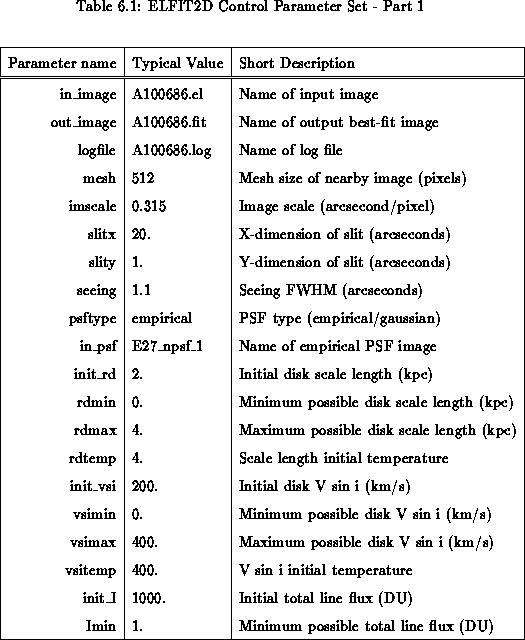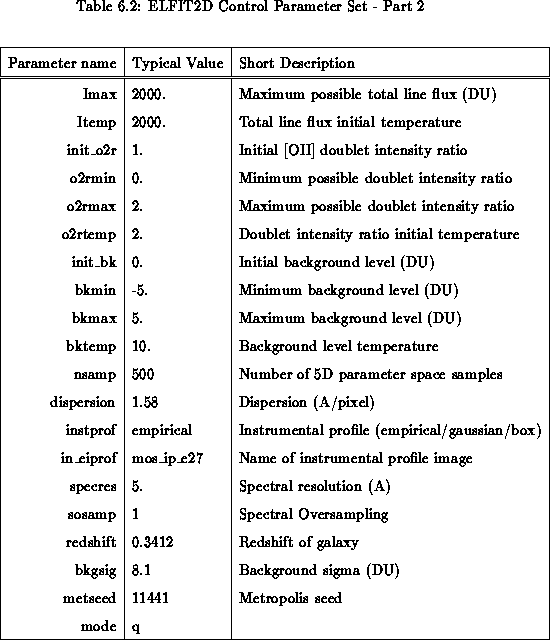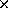ELFIT2D: 2D Emission Line FittingNext: Simulations Up: Synthetic Rotation Curve Previous: An Example: The

ELFIT2D: 2D Emission Line Fitting

This section describes the ELFIT2D program used to analyze low S/N spatially-resolved spectra of intermediate redshift galaxies. ELFIT2D was written by the author in the Subset Pre-Processor (SPP) language. SPP is the native language of IRAF. This choice was motivated by the flexibility in parameter management and image manipulations offered by SPP within the IRAF environment. ELFIT2D is used in the same way as any other IRAF tasks. It has a set of control parameters shown in Tablesand.In_image is the name of an input 2D postage image of a spatially-resolved emission spectrum obtained following Section. Out_image is the name of an image of a synthetic rotation curve created by ELFIT2D from the median values of the parameter values. Out_image can be subtracted from In_image to study fit residuals. ELFIT2D sends all the information on accepted Metropolis iterations and final median parameter values to logfile.

ELFIT2D starts up by creating a square grid (with dimensions given by mesh) which oversamples the spatial scale of input 2D spectrum by a factor of 64 (88). The scale of this grid (kiloparsecs/pixel) is computed from the input spectrum image scale imscale and the galaxy redshift using the standard angular distance relation for q= 0.5. There are two choices for the telescope's point-spread-function (PSF): Gaussian and empirical. For a Gaussian PSF, the FWHM is specified by seeing. For an empirical PSF, in_psf specifies the name of a ``postage stamp'' image of the PSF derived from stars on direct images. In both cases, the PSF is mapped onto a 128128 grid, and so are the slit dimensions slitx and slity. The PSF is then normalized to a unit volume and Fourier transformed in preparation for seeing convolutions later on. Synthetic rotation curves are created over a meshmesh grid as described in Section. The flux is integrated to rebin the meshmesh grid to the 128128 grid. Since values of mesh used are always multiples of 128, no flux interpolation is needed, and potential flux errors are avoided. The seeing convolution of each velocity slice (see Section) was performed using the Fourier Convolution Theorem over the 128128 grid to speed up calculations.

For each of the 5 synthetic rotation curve parameters, ELFIT2D has 4 control parameters. For example, init_vs, vsimin, vsimax and vsitemp control what steps will be taken by the Metropolis algorithm. Initially, trial values will be uniformly generated between init_vs0.5vsitemp and init_vs + 0.5vsitemp. As the Metropolis algorithm proceeds, both the temperature and the location around which steps are generated change, but trial values are never allowed outside the range defined by vsimin and vsimax. The value of a given parameter can be held fixed throughout a Metropolis search by simply setting its initial temperature to zero.

The Metropolis search stops after it has accepted nsamp iterations. The parameter dispersion gives the number of Angstroms per pixel in the input spectrum. The dispersion is set by the grism used during the observations. There are three choices for the instrumental profile of the spectrograph: gaussian, box and empirical. Obviously, the last choice is the best one to use when an instrumental profile is available. The parameter in_eiprof is the name of an 1D image containing an instrumental profile which can be derived from comparison arc lines for example (see Sectionfor details). Each column of the simulated 2D spectra was convolved with this 1D instrumental profile using the Fourier Convolution Theorem. End effects were avoided with zero-padding. The parameters specres and sosamp are only used for the gaussian and box instrumental profiles.

Finally, bkgsig is the standard deviation of background pixel values measured by performing image statistics in background sections of the input spectrum images, and metseed is a large odd integer number needed to seed the random generator used for the Metropolis trial values. The use of ELFIT2D is not restricted to the [OII] doublet emission line. Other emission lines can be easily analyzed by changing the emission line rest wavelength(s) specified in the first lines of the source code.

The performance of ELFIT2D is analyzed in the next section.Next: Simulations Up: Synthetic Rotation Curve Previous: An Example: The

Luc Simard
Mon Sep 2 12:37:40 PDT 1996